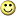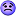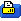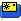Sep 03, 2012, 06:46 PM
Registered User
Discussion

# Spektrum DX8 and DX18 SD log file format

Hi

I have try to debug/hack the format of the Spektrum DX8 and DX18 SD log file SPEKTRUM.TLM and this is what I found:

Format of the SPEKTRUM.TLM file:

1 Time stamp Low byte
2 Time stamp
3 Time stamp
4 Time stamp high byte
5 Data type 00,16,17,7E,7F
6 ?

If Time stamp = FFFFFFFF then it is a header
Length of is 36 Byte
If 5 and 6 is equal then it is header Data
If 6 is 00 then it is name info

Then it repreat a 20 byte block:

1 Time stamp Low byte
2 Time stamp
3 Time stamp
4 Time stamp high byte
5 Data type 00,16,17,7E,7F

Data type = 00
?

Dat type = 16
1 serial number
2 serial number
3 serial number
4 always 00
5 16 (Data type)
6 always 00
7 not identified is low byte of next (decimal)
8 not identified is high byte of previous (decimal)
9 1/100th of a degree second latitude (decimal)
10 degree seconds latitude (decimal)
11 degree minutes latitude (decimal)
12 degrees latitude (decimal)
13 1/100th of a degree second longitude (decimal)
14 degree seconds longitude (decimal)
15 degree minutes longitude (decimal)
16 degrees longitude (decimal)
17 1/10th of degree heading (decimal)
18 degrees heading first two numbers (decimal)
19 always 08
20 always 3B

so to get decimal degrees calculate:

latitude = {12} + {11}/60 + ( {10} + {9}/100)/3600
longitude = {16}+ {15}/60 + ({14} + {13}/100)/3600

Offset to google maps seems to be
lat= lat-0,003655 lon= lon-0,004572

Data type = 17
6 ?
7 Speed High
8 Speed Low
9 ? May be max speed High
10 ? May be max speed Low
11 ?
12 ?
13 ?
14 ?
15 ?
16 ?
17 ?
18 ?
19 ?
20 ?

Data type = 18
6 ?
7 Altitude High
8 Altitude Low the format is in intiger and is in 0.1m
9 ?
10 ?
11 ?
12 ?
13 ?
14 ?
15 ?
16 ?
17 ?
18 ?
19 ?
20 ?

Data type = 20
6 ?
7 x High
8 x Low
9 y High
10 y Low
11 z High
12 z Low
13 x max High
14 x max Low
15 y max High
16 y max Low
17 z max High
18 z max Low
19 z min High
20 z min Low

Data type = 126
6 ?
7 RPM High
8 RPM Low RPM = (RPM low + (RPM High * 256)) * count of Poles
9 Volt High
10 Volt Low V = (Volt low + (Volt High * 256)) / 100
11 Temp High
12 Temp Low F = Temp low + (Temp high * 256) C = ((Temp low + (Temp high * 256)) - 32) / 1,8
13 ?
14 ?
15 ?
16 ?
17 ?
18 ?
19 ?
20 ?

Data type = 127
6 ?
7 A High
8 A Low
9 B High
10 B Low
11 L High
12 L Low
13 R High
14 R Low
15 Frame loss High
16 Frame loss Low
17 Holds High
18 Holds Low
19 Tranciver Volt High
20 Tranciver Volt Low V = (Tranciver Volt Low + (Tranciver Volt High * 256)) / 100

Br.
Kim
Last edited by KimDK; Oct 15, 2012 at 04:27 PM.
 Sign up now to remove ads between postsSep 04, 2012, 05:53 AM Registered User Thread OP Update: Data type = 18 6 ? 7 Altitude High 8 Altitude Low the format is in intiger and is in 0.1m 9 ? 10 ? 11 ? 12 ? 13 ? 14 ? 15 ? 16 ? 17 ? 18 ? 19 ? 20 ?
 Sep 04, 2012, 05:55 AM Registered User Thread OP I have made this VBA code to import logdata to Excel. You need to update RPM_poles! Code: ```Sub Import_Spektrum_log() Dim intFileNum% Dim bytTemp As Byte Dim intCellRow As Long Dim dlgSaveAs As FileDialog Dim SourceDir As String Dim DataMode As Integer Dim DataByte As Integer Dim DataByteToRead As Integer Dim DataTime(4) As Long Dim Speed As Long Dim MaxSpeed As Long Dim RPM As Long Dim RPM_Max As Long Dim RPM_poles As Long Dim Volt As Single Dim TempC As Single Dim RxVolt As Single Dim Altitude As Single Dim AltitudeInt As Long Dim AltitudeSign As Long Dim Name As String Dim Current As Single intFileNum = FreeFile intCellRow = 1 Set dlgSaveAs = Application.FileDialog(msoFileDialogOpen) ' Update the file name!!!! SourceDir = "F:\kim\rc\DX8\sd_log" dlgSaveAs.InitialFileName = SourceDir dlgSaveAs.AllowMultiSelect = False dlgSaveAs.Filters.Clear dlgSaveAs.Filters.Add "Spektrum log data", "*.tlm", 1 dlgSaveAs.FilterIndex = 1 'dlgSaveAs. dlgSaveAs.Show If dlgSaveAs.SelectedItems.Count = 1 Then SourceDir = dlgSaveAs.SelectedItems(1) Open SourceDir For Binary Access Read As intFileNum Else Exit Sub End If ' Read data intCellRow = 1 ' Reset controls DataMode = 0 DataByte = 0 DataByteToRead = 20 ' Reset info DataTime(1) = 0 DataTime(2) = 0 DataTime(3) = 0 DataTime(4) = 0 Speed = 0 MaxSpeed = 0 RPM = 0 RPM_Max = 65000 RPM_poles = 12 ' update this to match your flight Volt = 0 TempC = 0 RxVolt = 0 Altitude = 0 AltitudeInt = 0 AltitudeSign = 0 Name = "" Current = 0 Do While (Not EOF(intFileNum)) And intCellRow <= 100000 Get intFileNum, , bytTemp DataByte = DataByte + 1 Select Case DataByte Case 1 ' Time Low DataTime(1) = bytTemp Case 2 ' Time DataTime(2) = bytTemp Case 3 ' Time DataTime(3) = bytTemp Case 4 ' Time High DataTime(4) = bytTemp If (DataTime(1) = 255) And (DataTime(2) = 255) And (DataTime(3) = 255) And (DataTime(4) = 255) Then DataTime(0) = 0 DataMode = -1 DataByteToRead = 36 Else DataTime(0) = DataTime(1) DataTime(0) = DataTime(0) + (DataTime(2) * CLng(256)) DataTime(0) = DataTime(0) + (DataTime(3) * CLng(256) * CLng(256)) DataTime(0) = DataTime(0) + (DataTime(4) * CLng(256) * CLng(256) * CLng(256)) DataByteToRead = 20 End If Case 5 ' Mode If DataMode <> -1 Then DataMode = bytTemp End If Case 6 To 36 Select Case DataMode Case -1 ' Header Select Case DataByte Case 6 Name = "" If bytTemp = 0 Then DataMode = -2 End If Case Else End Select Case -2 ' Header Select Case DataByte Case 13 To 22 Name = Name & Chr(bytTemp) Case Else End Select Case 3 ' Current Select Case DataByte Case 6 Current = 0 Case 7 Current = bytTemp * CLng(256) Case 8 Current = Current + bytTemp Current = Current * 0.1976 Case Else End Select Case 17 ' Speed Select Case DataByte Case 6 Speed = 0 MaxSpeed = 0 Case 7 Speed = bytTemp * CLng(256) Case 8 Speed = Speed + bytTemp Case 9 MaxSpeed = bytTemp * CLng(256) Case 10 MaxSpeed = Speed + bytTemp Case Else End Select Case 18 ' Altitude Select Case DataByte Case 6 Altitude = 0 AltitudeInt = 0 Case 7 AltitudeInt = bytTemp * CLng(256) Case 8 AltitudeInt = AltitudeInt + bytTemp AltitudeSign = AltitudeInt And &H8000 AltitudeInt = AltitudeInt And &H7FFF If AltitudeSign = 32768 Then Altitude = AltitudeInt - 32767 Else Altitude = AltitudeInt End If Altitude = Altitude / 10 If (Altitude > 1000) Or (Altitude < -1000) Then Altitude = 0 End If Case Else End Select Case 126 ' RPM,Volt,Temp Select Case DataByte Case 6 RPM = 0 Volt = 0 TempC = 0 Case 7 RPM = bytTemp * CLng(256) Case 8 RPM = RPM + bytTemp If (RPM = 65535) Or (RPM < 200) Then RPM = 0 Else RPM = (120000000 / RPM_poles) / RPM End If Case 9 Volt = bytTemp * CLng(256) Case 10 Volt = Volt + bytTemp Volt = Volt / 100 If (Volt > 100) Or (Volt < -100) Then Volt = 0 End If Case 11 TempC = bytTemp * CLng(256) Case 12 TempC = TempC + bytTemp TempC = (TempC - 32) / 1.8 If TempC > 500 Or TempC < -100 Then TempC = 0 End If Case Else End Select Case 127 ' Radio,RxVolt Select Case DataByte Case 6 RxVolt = 0 Case 19 RxVolt = bytTemp * CLng(256) Case 20 RxVolt = RxVolt + bytTemp RxVolt = RxVolt / 100 Case Else End Select Case Else End Select Case Else DataMode = 0 DataByte = 0 End Select ' Print data If DataByte >= DataByteToRead Then If DataMode = 127 Then intCellRow = intCellRow + 1 Cells(intCellRow, 1) = DataTime Cells(intCellRow, 2) = Speed Cells(intCellRow, 3) = MaxSpeed Cells(intCellRow, 4) = RPM Cells(intCellRow, 5) = Volt Cells(intCellRow, 6) = TempC Cells(intCellRow, 7) = RxVolt Cells(intCellRow, 8) = Altitude Cells(intCellRow, 9) = Current End If If DataMode = -2 Then ' Nyt ark Sheets.Add After:=Sheets(Sheets.Count) Sheets(Sheets.Count).Name = Sheets(Sheets.Count).Name & " " & Name Cells.Select Selection.ClearContents Columns("A:A").Select Selection.NumberFormat = "0" Columns("B:B").Select Selection.NumberFormat = "0" Columns("C:C").Select Selection.NumberFormat = "0" Columns("D:D").Select Selection.NumberFormat = "0" Columns("E:E").Select Selection.NumberFormat = "0.00" Columns("F:F").Select Selection.NumberFormat = "0.0" Columns("G:G").Select Selection.NumberFormat = "0.00" Columns("H:H").Select Selection.NumberFormat = "0.0" Columns("I:I").Select Selection.NumberFormat = "0.00" Cells(1, 1) = "Time stamp" Cells(1, 2) = "Speed" Cells(1, 3) = "MaxSpeed" Cells(1, 4) = "RPM" Cells(1, 5) = "Volt" Cells(1, 6) = "TempC" Cells(1, 7) = "RxVolt" Cells(1, 8) = "Altitude" Cells(1, 9) = "Current" ' Read data intCellRow = 1 ' Reset controls DataByteToRead = 20 ' Reset info DataTime(1) = 0 DataTime(2) = 0 DataTime(3) = 0 DataTime(4) = 0 Speed = 0 MaxSpeed = 0 RPM = 0 Volt = 0 TempC = 0 RxVolt = 0 Altitude = 0 AltitudeInt = 0 AltitudeSign = 0 Current = 0 End If DataMode = 0 DataByte = 0 End If Loop Close intFileNum End Sub``` Last edited by KimDK; Sep 04, 2012 at 10:31 AM.
 Sep 04, 2012, 06:20 AM Surface, Air & Water Rc Toys.. Interesting, are you working on a program of some sort?
 Sep 04, 2012, 07:37 AM Registered User Thread OP No, not a program, but i need a way to read the log with out using iOS device. And Excel can make the view I need. Thats way I made the VBA script to read the file. If there is some having SPEKTRUM.TLM files with info from other sensor then Speed, RPM, Volt, Temp, RxVolt, Altitude then pleas upload them and i will try to find the information and add them to the script.
 Sep 04, 2012, 10:19 AM Registered User I think I can provide some more info from the other side. I currently play around with the TM1000 side to substitute various sensorsThe data is gathered from the sensors with an I�C interface. 16 bytes, the first is the device, second is 00. Then 14 bytes of data.. 03: Current. (A) 00 MSB LSB and the rest is 00 one unit of current is 0.1976A. A little bit strange, seems to be related to the used current sensor. Adress 0a is Powerbox. I will post the rest the next days when I am finished hackingMaybe you can create a converter to geht the data into LogView ?
 Sep 04, 2012, 10:30 AM Registered User Thread OP Thangs for the infoI have update the import function to devide the logs.
 Sep 04, 2012, 03:32 PM Registered User Thread OP When import to excel it can look like this when make a diagram.
 Sep 04, 2012, 04:11 PM Surface, Air & Water Rc Toys.. Nice, almost looked like I was looking at my CC Ice log files. Keep up the good work, I am sure many will be interested.
 Sep 04, 2012, 05:03 PM Registered User Great job.....
Sep 06, 2012, 06:11 AM
Registered User
Quote:
 Originally Posted by faxxe great job.....
+1Subscribed.
Last edited by egicar; Sep 06, 2012 at 06:18 AM.
Sep 06, 2012, 06:05 PM
Registered User

# First version of a converter to LogView

Hi

Hir is my first version of a converter to convert Spektrum log file (SPEKTRUM.TLM) to a LogView format.

When more ready I will also provide the source code.

Kim

### Files

Last edited by KimDK; Sep 07, 2012 at 12:41 PM.
Sep 07, 2012, 12:46 PM
Registered User

# SPEKTRUM.TLM files for test

Hi

I need SPEKTRUM.TLM files to test my program pleas upload if you have one that have other sensor then Speed, RPM, Volt, Temp, RxVolt, Altitude.
 Sep 09, 2012, 09:48 AM Registered User Thread OP I was out flying to day, and this is a log of a takeoff.
 Sep 10, 2012, 02:20 AM Registered User I'm sorry: I don't have a rich TLM file: I have the TM1000/1100 stock sensors. You are making a very good work!!!Maybe, we need some more instructions about using your program. Here is what I did:I opened my Spektrum.TLM file and saved the CVS files in a directrory I launched LogView and imported the CVS I saved the file in LogView format (LOV) Is this the right way? Ciao, Egidio

 Thread ToolsShow Printable VersionEmail this Page